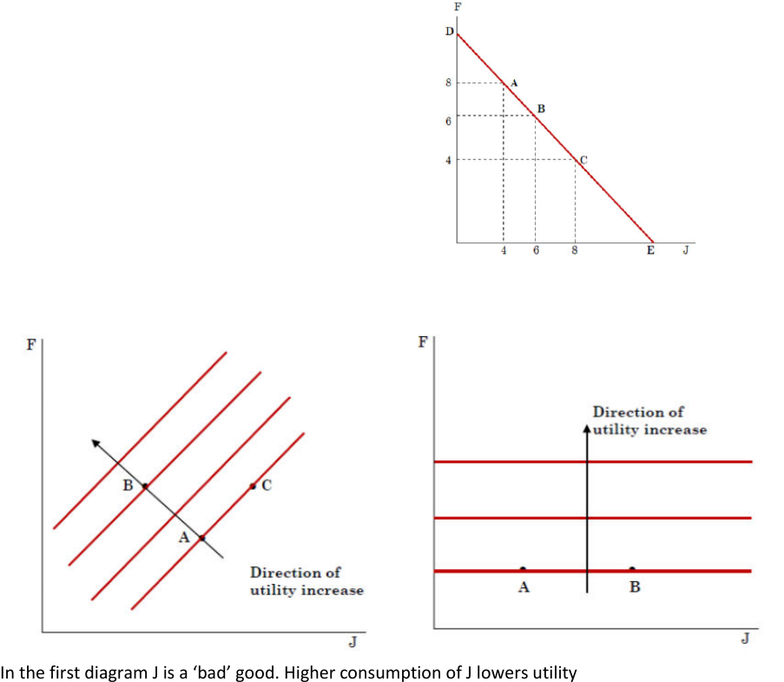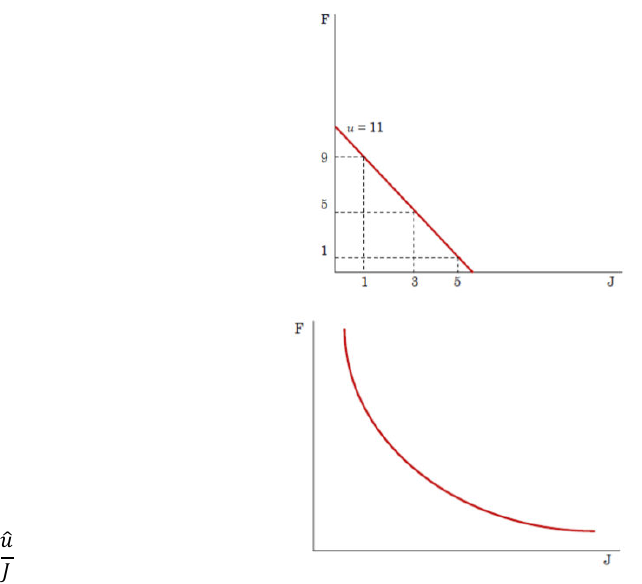Get 2 days of unlimited access
Class Notes (1,000,000)
AUS (30,000)
UniMelb (5,000)
Economics (200)
Lecture 4

# ECON20002 Lecture 4: Week 2 Lecture 2

Department
Economics
Course Code
ECON20002
Professor
Lecture
4

This preview shows page 1. to view the full 5 pages of the document.Week 2 Lecture 2
Perfect Substitutes
Our four assumptions all hold concurrently for “well-behaved” preferences
But consider the case below
here, the MRS of J for F is constant at all times
When this is the case, we say that J and F are perfect
substitutes
Discussion
Why does the substitutability between goods
matter?
How substitutable two goods are will affect to what
extent an increase in the price of good 1 will raise
the demand for good two.
Non-Standard Indifference Curves
In the first diagram J is a ‘badgood. Higher consumption of J lowers utility
In the second diagram J is a ‘neutral’ good. Higher consumption of J does not affect utility
Utility
Mathematically, it is useful to assign numerical values to represent the level of satisfaction
We do this using a concept known as utility
We can use a utility function to assign utility values to various market baskets
This function is an alternative way of representing the preferences of a consumer
For example, the following is an example of a utility function
𝑢(𝐽,𝐹)= 2𝐽 +𝐹
Given the function, we know that a basket consisting of one unit of J and one unit of F will
have a utility value of
###### You're Reading a Preview

Unlock to view full version

Only page 1 are available for preview. Some parts have been intentionally blurred.𝑢(1,1)=(2×1)+1 = 3
Similarly 𝑢(1,2)= 4 and 𝑢(2,3)= 7 and so on
A utility function must have the following properties
o 𝑢(𝐴)> 𝑢(𝐵) whenever 𝐴 > 𝐵
o 𝑢(𝐴)= 𝑢(𝐵) whenever 𝐴~𝐵
Utility does not have any natural or physical units
We can use utility to rank different baskets. However, we cannot use it to say how much one
basket is preferred to another
This is similar to the information provided by your grade for the subject (e.g. H1, H2A and so
forth)
Thus, just like your grade, utilities are ordinal numbers
On the other hand, the mark you receive for this subject is a cardinal number
Important: a utility function provides an ordinal rank of different baskets. It does not tell us
by how much one basket is preferred to another.
Utility Functions and Indifference Curves
Suppose the utility function is:
𝑢(𝐽,𝐹)= 2𝐽 +𝐹
To sketch this consumer’s indifference curve, we can
find various baskets of J and F that yield the same utility
For example, the basket (1,9) (3,5) and (5,1) all y9ield
𝑢 = 11. We can use this to draw an indifference curve
Notice that this is a utility function where J and F are
perfect substitutes
Now consider a more general case where the utility
function is as follows:
𝑢 = 𝐽 ×𝐹
To sketch this consumer’s indifference curve, we need
to fix utility at some level 𝑢̂
We can then rewrite the above to get the following
expression
𝐹 = 𝑢̂
𝐽
Marginal Utility
The concept of utility allows us to think of a consumer’s marginal rate of substitution in a
new way
Before doing so, lets first review some terminology
Total utility: utility obtained from total consumption of a good
Marginal utility: utility obtained from consuming one additional unit of a good, or:
###### You're Reading a Preview

Unlock to view full version